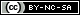# Weibull++ Examples

(Difference between revisions)
 Revision as of 15:34, 13 June 2014 (view source)← Older edit Revision as of 21:11, 24 June 2015 (view source) (→Parametric Life Data Analysis)Newer edit → Line 18: Line 18: *Simple Probability Plotting Examples: [[Probability Plotting Example|Simple Example]], [[3-Parameter_Weibull_Example|3P-Weibull Example]], [[1P_Exponential_Example|1P-Exponential]], [[Normal Distribution Probability Plotting Example|Normal]], [[Example:_Lognormal_Distribution_Probability_Plot|Lognormal]] *Simple Probability Plotting Examples: [[Probability Plotting Example|Simple Example]], [[3-Parameter_Weibull_Example|3P-Weibull Example]], [[1P_Exponential_Example|1P-Exponential]], [[Normal Distribution Probability Plotting Example|Normal]], [[Example:_Lognormal_Distribution_Probability_Plot|Lognormal]] *[[Standard_Folio_Plots|Standard Folio Plots]] *[[Standard_Folio_Plots|Standard Folio Plots]] - *Examples by Distribution: - :*[[Weibull Distribution Examples|Weibull]] - :*[[Exponential Distribution Examples|Exponential]] - :*[[Normal Distribution Examples|Normal]] - :*[[Logistic_Distribution_Example|Logistic]] - :*[[Loglogistic_Distribution_Example|Loglogistic]] - :*[[Gamma_Distribution_Example|Gamma]] - :*[[Generalized_Gamma_Distribution_Example|Generalized Gamma ]] - :*[[Gumbel_Distribution_Example|Gumbel]] - *[[Bayesian-Weibull_Lognormal_Prior Example|Bayesian-Weibull Analysis]] - *[[Mixed Weibull Example]] * Competing Failure Modes Analysis * Competing Failure Modes Analysis :* Two Failure Modes Example. View it in '''[http://www.reliasoft.com/Weibull/examples/rc10/index.htm HTML]''' or        '''[http://www.reliasoft.tv/weibull/appexamples/weibull_app_ex_10.html Video]''' :* Two Failure Modes Example. View it in '''[http://www.reliasoft.com/Weibull/examples/rc10/index.htm HTML]''' or        '''[http://www.reliasoft.tv/weibull/appexamples/weibull_app_ex_10.html Video]'''

## Quick Start Guide

The Weibull++ & ALTA Quick Start Guide has been designed to help you explore many of the software's key features by working through step-by-step instructions for some practical application examples. The guide is available as a free *.PDF download and you can also watch videos posted on ReliaSoft.tv.

## Non-Parametric Life Data Analysis

• Crack Propagation Example (Point Estimation). View it in HTML or Video

## UtilitiesContent on this site is available/licensed under the "Creative Commons Attribution-NonCommercial-ShareAlike 4.0 International License." See Copyright Information for details.